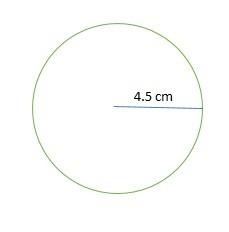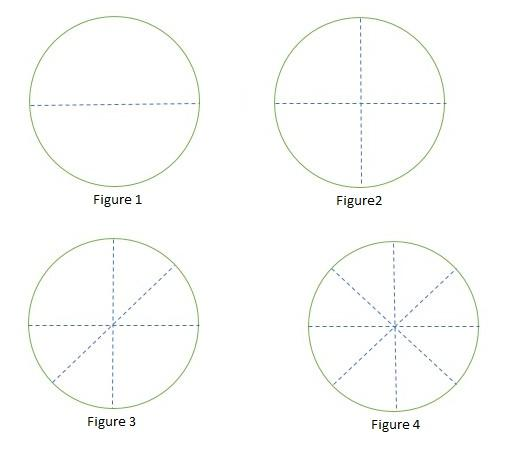QUESTION

# Draw a circle of 4.5 cm radius & divide the circle into 8 equal parts.

Hint: First of all, construct a circle with the given radius of the circle. Then cut into 8 equal parts by dividing the central angle into 8 equal parts. By drawing all the diameter. we will get the circle into 8 equal parts.So the diameter of the circle $= 2 \times 4.5 = 9{\text{ cm}}$
Step 3: Draw a line making an angle of ${45^0}$ with the horizontal line of length 9 cm on the circle as shown in figure 3.
Step 4: Draw a line making an angle of ${135^0}$ with the horizontal line of length 9 cm on the circle to divide into 8 equal parts as shown in figure 4.Note: The diameter of the circle is equal to twice the length of radius of the circle. The area of each part is equal to $\dfrac{1}{8}$ part of the circle. If you notice here, we have also shown how to divide the circle into 2 equal parts and 4 equal parts of radius 4.5 cm.